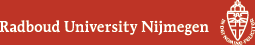## Geometry Seminar - Abstracts

### Talk

Tuesday 25 June 2019, 16:00-17:00 in HG00.308
Matthias Lienert (Tübingen)
Multi-time wave equations

### Abstract

The basic object in quantum physics is a wave function which usually depends on one time variable and $$N$$ space variables for $$N$$ particles. However, such an object is not covariant under the Poincare group, the basic symmetry group of (special) relativity. A straightforward generalization which fixes this problem is to let the wave function $$\psi = \psi(x_1,...,x_N)$$ depend on one spacetime variable $$x_i$$ per particle. Because of the presence of $$N$$ time variables $$t_i = x_i^0, i = 1,2,...,N$$ $$\psi$$ is called a "multi-time wave function". As natural as this concept may sound, one is led to a range of challenging physical and mathematical problems, such as: How can one define a consistent and interacting time evolution in the multiple time $$(x_i^0)$$ variables? Which types of PDEs work for that? What is the physical meaning of $$|\psi|^2$$ at unequal times?, and: Are there new possibilities for evolution equations which are specific to the multi-time formalism? This talk will provide a non-technical overview of recent progress concerning these questions.

(Back to geometry seminar schedule)